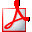Uniwersytet Warszawski - Centralny System Uwierzytelniania Nie jesteś zalogowany | zaloguj sięDrukuj sylabus

# IT TOOLS IN ECONOMICS

## Informacje ogólne

 Kod przedmiotu: 2400-PP1NIa Kod Erasmus / ISCED: 14.3/ (0311) EkonomiaNazwa przedmiotu: IT TOOLS IN ECONOMICS Jednostka: Wydział Nauk Ekonomicznych Grupy: Przedmioty obowiązkowe dla I r. licencjackich : Ekonomia, specjalność: MSEMen Przedmioty obowiązkowe dla I r. licencjackich Międzykierunkowych Studiów Ekonomiczno-Matematycznych Przedmioty obowiązkowe dla I r. studiów licencjackich (Ekonomia) - program podstawowy Punkty ECTS i inne: 3.00zobacz reguły punktacji Język prowadzenia: angielski Rodzaj przedmiotu: obowiązkowe Założenia (opisowo): (tylko po angielsku) Basic IT tools knowledge gained in secondary school, knowledge of the mathematics gained during Linear Algebra course and dirst part of Mathematical Analysis course Skrócony opis: The goal of this course is to present participants basics of IT technology applied in everyday work of an economist. During laboratories students will achieve knowledge and skills of using selected IT tools (advanced Excel, Matlab, Mathematica) and applying appropriate tool to certain economic problems solving. Participation in the course requires basic knowledge of IT and mathematics (scope of secondary school and courses of Linear Algebra and Mathematical Analysis held in the first semester) and ability of using Excel spreadsheet. Knowledge gained by students during this course will be assessed with help of one mid-term test and final test. Pełny opis: 1) Introduction to performing advanced economic computations with help od IT tools 2) Extending Excel knowledge: loading data from external sources, data sorting and filtering, relative and absolute cells addressing, application of computational formulas and use of pivot tables and charts 3) Finding roots of nonlinear equations of one variable: 4) Using macros 5) Introduction to Mathematica - using expressions and matrices, access to help system, access to external data sources 6) Introduction to Matlab - using expressions and matrices, access to help system, access to external data sources 7) Graphics functions, reports generation, storing results of computations in Mathematica and Matlab 8) Computing limits of sequences and examining asymptotic characteristic of series 9) Symbolic matrix transformations - computing determinant, inverse, eigenvalues and eigenvectors of a matrix. solving systems of linear equations 10) Symbolic computations of derivatives and integrals of univariate and multivariate functions 11) Mid-term test 12) Numerical matrix transformations - computing determinant, inverse, eigenvalues and eigenvectors of a matrix, solving systems of linear equations; Comparing results with symbolic computations 13) Numerical derivatives computation (backward and forward differences method) and integral computation (trapezoid method and Simpson method) 14) Gradient constrained and unconstrained optimization methods of uni- and multivariate functions Literatura: Elements of Mathematica and Matlab help systems Abell M., Braselton J., Mathematica by Example, 4th Edition, Elsevier, 2009 Dodge M., Stinson C., Running Microsoft Excel 2000, Microsoft Press, 1999 Gilat A., MATLAB: An Introduction with Applications, Willey, 2008 Peal D., Excel 2003, Top 100 Simplified, Willey, 2003 Walkenbach J., Excel 2003 Bible, Willey, 2003 Efekty uczenia się: Upon completion of this course student will be able to: - Assess purposefulness of particular mathematical schema application for solving certain problem from domain of economics - Choose appropriate IT tool (Excel, Mathematica, Matlab) to apply selected mathematical schema for solving the problem in practice and represent appropriately this problem in selected tool - Perform sequence of operations aimed at gaining valid results - Conduct analysis of achieved results and interpret its economic meaning KU03 Metody i kryteria oceniania: Knowledge and skills of students participating in this course will be assessed during - Mid-term test organized after completion of Excel and Mathematica modules - Final test The main goal of the mid-term test will be to assess knowledge and skills of students achieved in the first part of the course during modules held with Excel and Mathematica. Second aim of this test will be to show students possible fields of improvements before final test. Mid-term test will consist of two problems embracing topics covered by the lecturer during the first part of the course. Problems are supposed to be solved with help of a computer with appropriate software. Main criteria for the assessment will be correctness and conciseness of the solution. Final test will comprise of 4 problems, which are supposed to be solved with help of Excel, Mathematica and Matlab in the front of a computer.

## Zajęcia w cyklu "Semestr letni 2021/22" (w trakcie)

 Okres: 2022-02-21 - 2022-06-15Wybrany podział planu: ten tydzień cykl przedmiotuzobacz plan zajęć Typ zajęć: Konwersatorium, 30 godzinwięcej informacji Koordynatorzy: Karolina Kuligowska Prowadzący grup: Umair Ashraf Rana Lista studentów: (nie masz dostępu) Zaliczenie: Przedmiot - Zaliczenie na ocenę Konwersatorium - Zaliczenie na ocenę
Opisy przedmiotów w USOS i USOSweb są chronione prawem autorskim.
Właścicielem praw autorskich jest Uniwersytet Warszawski.